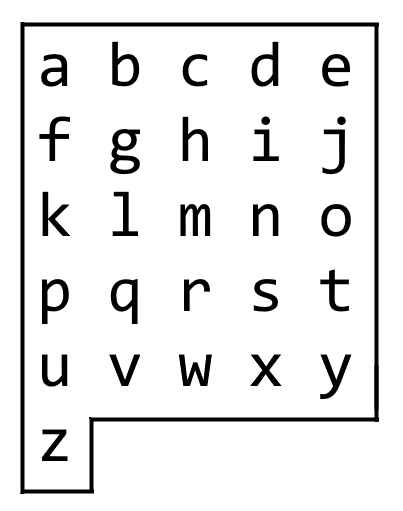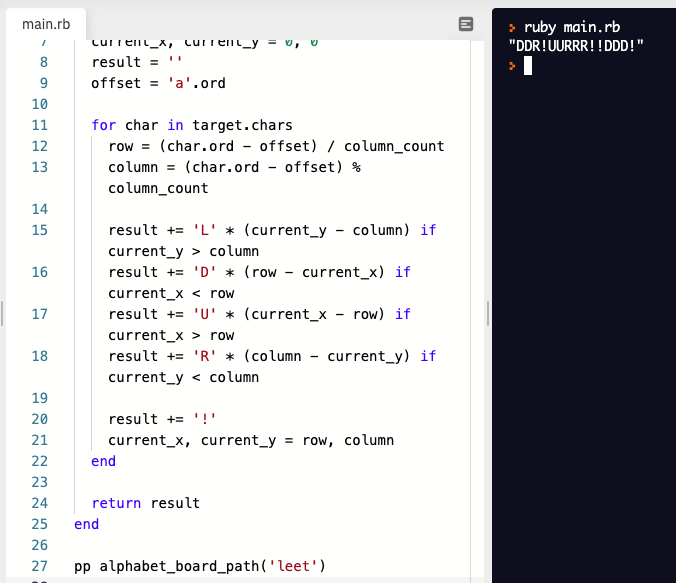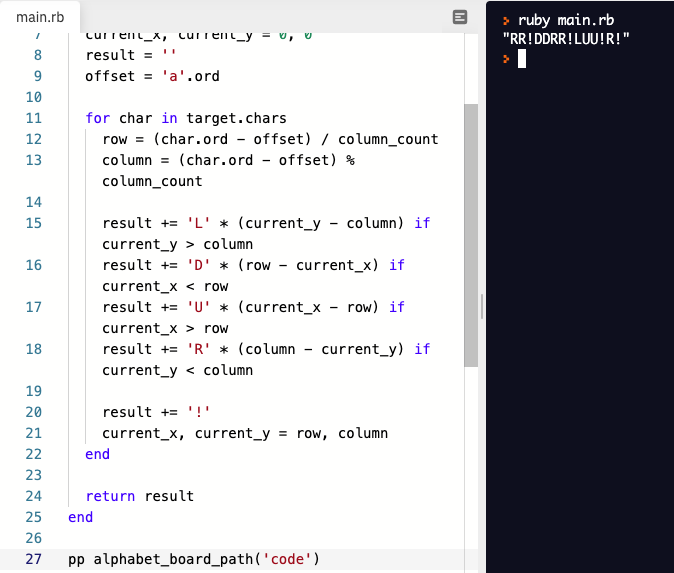# Stan Blog

## 學習過程中的一些記錄

#### 題目 轉自 leetCode

On an alphabet board, we start at position `(0, 0)`, corresponding to character `board`.

Here, `board = ["abcde", "fghij", "klmno", "pqrst", "uvwxy", "z"]`, as shown in the diagram below.We may make the following moves:

```````'U'` moves our position up one row, if the position exists on the board;
`'D'` moves our position down one row, if the position exists on the board;
`'L'` moves our position left one column, if the position exists on the board;
`'R'` moves our position right one column, if the position exists on the board;
`'!'` adds the character `board[r][c]` at our current position `(r, c)` to the answer.
(Here, the only positions that exist on the board are positions with letters on them.)
``````

Return a sequence of moves that makes our answer equal to `target` in the minimum number of moves. You may return any path that does so.

``````Example 1:

Input: target = "leet"
Output: "DDR!UURRR!!DDD!"

Example 2:

Input: target = "code"
Output: "RR!DDRR!UUL!R!"
``````

#### 解題思路

1. 找出每個字元的位置 `row、column`
2. 以目前座標，往目標位置移動
3. 找到該字元，以 `!` 做選擇

#### 範例程式碼

##### Ruby 範例

``````board = ["abcde", "fghij", "klmno", "pqrst", "uvwxy", "z"]

# @param {String} target
# @return {String}
def alphabet_board_path(target)
column_count = 5
current_x, current_y = 0, 0
result = ''
offset = 'a'.ord

for char in target.chars
row = (char.ord - offset) / column_count
column = (char.ord - offset) % column_count

result += 'L' * (current_y - column) if current_y > column
result += 'D' * (row - current_x) if current_x < row
result += 'U' * (current_x - row) if current_x > row
result += 'R' * (column - current_y) if current_y < column

result += '!'
current_x, current_y = row, column
end

return result
end

pp alphabet_board_path('leet')
``````

target 為 leet 時target 為 code 時#### Comments

comments powered by Disqus